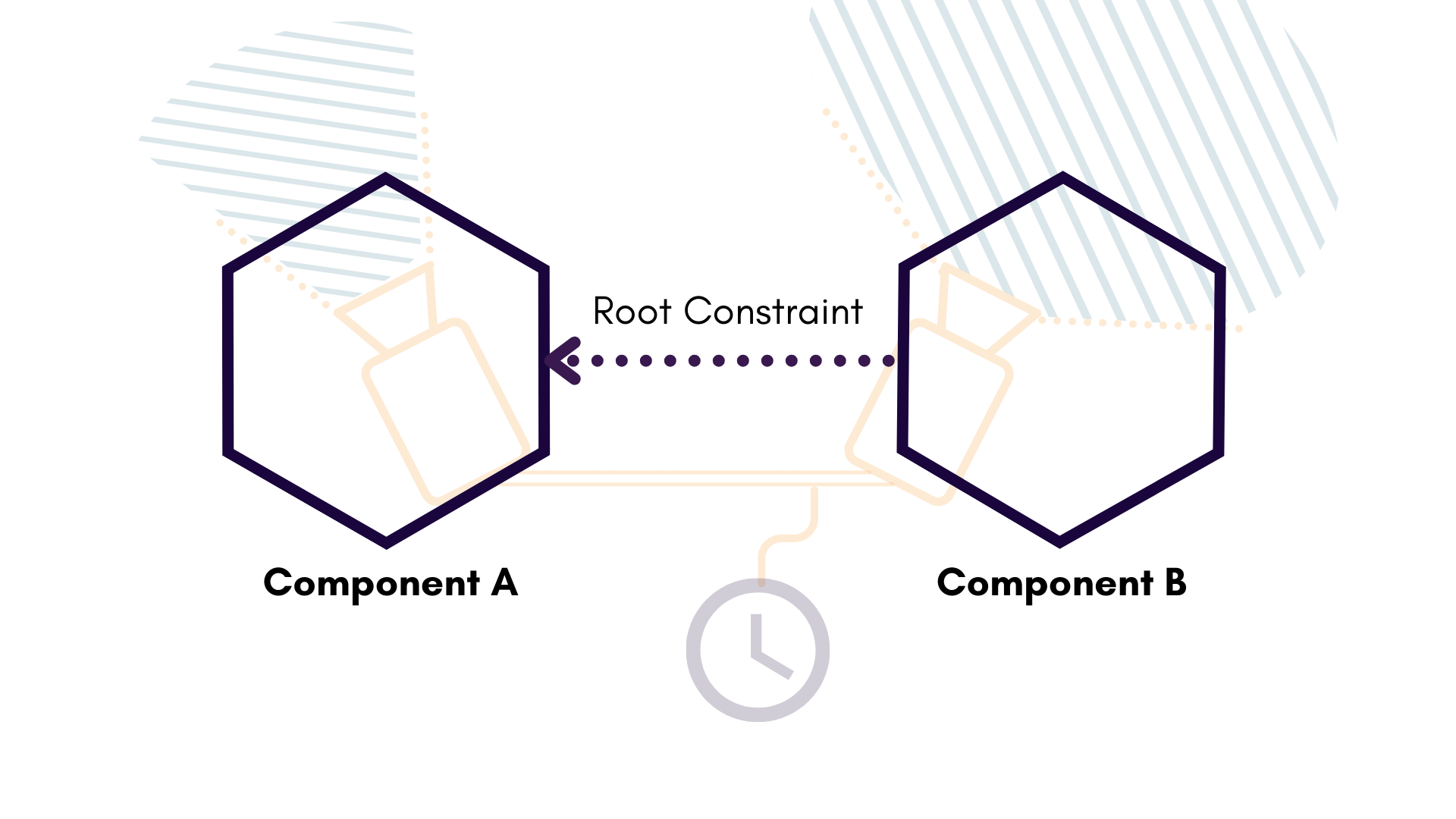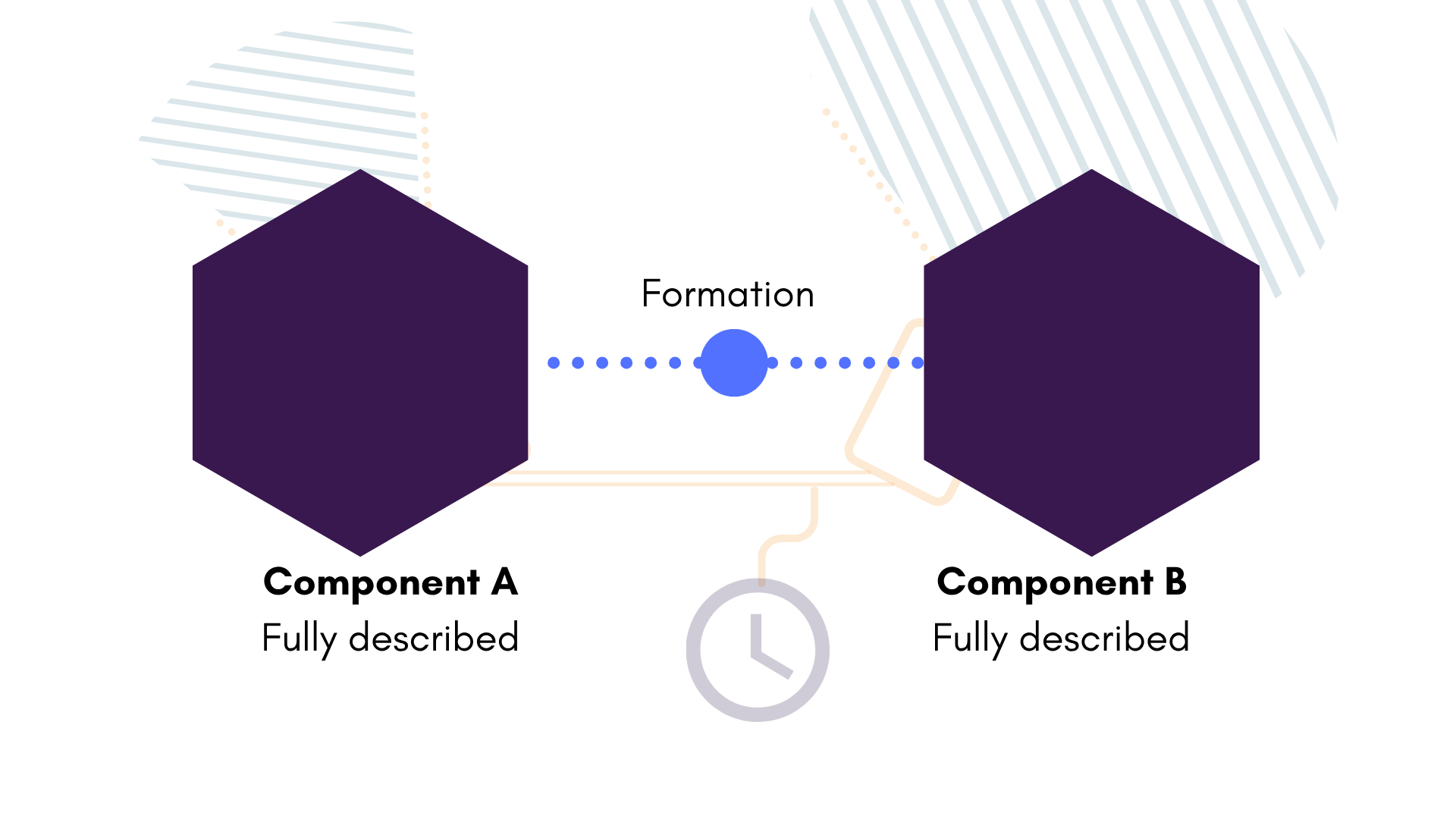## Designing the Root Constraint

The first thing to ask when creating a Plex is: where does our root constraint point? The root constraint acts as a symbolic links between related components in the Plex. It's purely for human use, but we find that it helps simplify Plex that would be otherwise complicated.

Constraint - Root

It's common to group components on the same circuitboard or clock with the same root. Here, we just have two cameras; either component will do. Let's choose component A.Now we can start building our first component, Camera A.

## Basic Camera Info

Component - Camera

Check out the Camera documentation for more info on this component type.

Some information on our camera is straightforward: its name, the size of its images, its pixel pitch. These things are mechanical and set. Let's note them first.

// The name of our camera. This should coincide with the data source
let cam_name = "alpha";

// VGA Image
let img_width = 640;
let img_height = 480;

// The pixel pitch of the camera. This is in meters per pixel
// (so it's usually quite small). If you don't know this number
// for a camera, we can default to 1, which will keep everything
// else in units of pixels.
let cam_pixel_pitch = 1.0;

// The unique identifier generated for this component
let camera_a_uuid = Uuid::new_v4();

# The name of our camera. This should coincide with the data source
cam_name = "alpha"

# VGA Image
width = 640
height = 480

# The pixel pitch of the camera. This is in meters per pixel
# (so it's usually quite small). If you don't know this number
# for a camera, we can default to 1, which will keep everything
# else in units of pixels.
cam_pixel_pitch = 1.0

# The unique identifier generated for this component
camera_a_uuid = uuid.uuid4()

// The name of our camera. This should coincide with the data source
std::string cam_name = "alpha";

// VGA Image
uint32_t width = 640;
uint32_t height = 480;

// The pixel pitch of the camera. This is in meters per pixel
// (so it's usually quite small). If you don't know this number
// for a camera, we can default to 1, which will keep everything
// else in units of pixels.
float cam_pixel_pitch = 1.0;

// The unique identifier generated for this component
uuids::uuid const cam_uuid = generate_uuid_v4();
std::string cam_uuid_str = uuids::to_string<char>(cam_uuid);


## Intrinsics

Now we fill in our camera model intrinsics. Unless we have calibrated our cameras before, we probably won't know these numbers off-hand. Not to worry; we can take an educated guess.

Since this camera has a narrow field-of-view lens, we should feel comfortable using Brown-Conrady distortion.

TVCal

We don't know those distortion numbers off-hand, but we could get them by running data from this system through TVCal!

let camera_a_intrinsics = {
// cx and cy are approximately in the centre of the image.
let cx = (img_width / 2).value_as::<f64>()?;
let cy = (img_height / 2).value_as::<f64>()?;

// This is approximately field of view of camera alpha from
let fov = 80;

// This is a rough approximation of the focal length based purely on the
// field-of-view of the lens.
let radius = (cx * cx + cy * cy).sqrt();
let f = radius / (field_of_view / 2.0).tan();

// Get our pinhole model down
let projection = ProjectionModel::Pinhole { f, cx, cy };

// Brown-Conrady distortion for a narrow FOV lens.
// Initialize to 0 for now.
k1: 0.0,
k2: 0.0,
k3: 0.0,
p1: 0.0,
p2: 0.0,
};

// This is a well-constructed camera, so no need for affinity
let affinity = AffinityModel::NoAffinity;

CameraIntrinsics {
projection,
distortion,
affinity,
width: img_width,
height: img_height,
}
};

# cx and cy are approximately in the centre of the image.
cx = width / 2
cy = height/2

# This is approximately field of view of the camera from corner-to-corner
# of the camera, in radians.
field_of_view = 80 * (math.pi / 180)

# This is a rough approximation of the focal length based purely on the
# field-of-view of the lens.
radius = math.sqrt(cx * cx + cy * cy)
f = radius / math.tan(field_of_view / 2.0)

# Get our pinhole model down
projection = plex.Projection(plex.Pinhole(cx, cy, f))

distortion = plex.Distortion(
None # Not using Kannala-Brandt, so set to None
)

intrinsics = plex.Intrinsics(
projection=projection,
distortion=distortion,
affinity=None, # This is a well-constructed camera, so no need for affinity
width=width,
height=height)

// cx and cy are approximately in the centre of the image.
double cx = width / 2;
double cy = height / 2;

// This is approximately field of view of the camera from corner-to-corner
// of the camera, in radians.
double field_of_view = 80 * (M_PI / 180);

// This is a rough approximation of the focal length based purely on the
// field-of-view of the lens.
double radius = sqrt(cx * cx + cy * cy);
double f = radius / tan(field_of_view / 2.0);

// Get our pinhole model down
tangviz::Pinhole pinhole{cx, cy, f};
tangviz::Projection projection{pinhole};

// Brown-Conrady distortion for a narrow FOV lens.
// Initialize to 0 for now.
0.0, 0.0, 0.0, 0.0, 0.0,
};
tangviz::Distortion distortion{
nullptr // No Kannala-Brandt here
};

tangviz::Intrinsics intrinsics{
nullptr, // This is a well-constructed camera, so no need for affinity
std::make_shared<tangviz::Distortion>(distortion),
height,
projection,
width
};


## Covariance

Camera intrinsics covariance gives us an idea of how certain (or uncertain) we are about the model numbers. It's good to provide these with realistic estimates; the numbers in the sample below should be good enough for many cases.

The Tangram Vision Platform is unique in that it provides meaningful covariance results from any calibration. Understanding these numbers can give great insight and control of any perception system. Learn more in the covariance documentation.

// Construct our covariance matrix. Covariance is always ordered
// - Projection
// - Distortion
// - Affinity
let camera_a_covariance = DMatrix::<f64>::from_diagonal(&DVector::from_iterator(
8,
vec![
100.0, // standard deviation of f
25.0,  // standard deviation of cx
25.0,  // standard deviation of cy
1.0,   // standard deviation of k1
1.0,   // standard deviation of k2
1.0,   // standard deviation of k3
1.0,   // standard deviation of p1
1.0,   // standard deviation of p2
]
.into_iter()
.map(|x: f64| x.powi(2)), // Variance is std. deviation squared
));

proj_covariance = [100.0,  # standard deviation of f
25.0,  # standard deviation of cx
25.0,  # standard deviation of cy
]

dist_covariance = [1.0,  # standard deviation of k1
1.0,  # standard deviation of k2
1.0,  # standard deviation of k3
1.0,  # standard deviation of p1
1.0,  # standard deviation of p2
]

# Construct our covariance matrix. Covariance is always ordered
# - Projection
# - Distortion
# - Affinity
covariance_diag = proj_covariance + dist_covariance

# Convert this from std_dev to variance by squaring it
covariance_diag = np.square(covariance_diag)

# A plex takes covariance in the format
#   [[flattened covariance matrix], matrix len, matrix len]
# This is a quirk of nalgebra's serialization (a Rust mathematics crate)
covariance = np.zeros((len(covariance_diag), len(covariance_diag)), float)
np.fill_diagonal(covariance, covariance_diag)
covariance_formatted = [covariance.flatten().tolist(), len(
covariance_diag), len(covariance_diag)]

std::vector<double> proj_covariance = std::vector<double>{
100.0, // standard deviation of f
25.0,  // standard deviation of cx
25.0   // standard deviation of cy
};
std::vector<double> dist_covariance = std::vector<double>{
1.0, // standard deviation of k1
1.0, // standard deviation of k2
1.0, // standard deviation of k3
1.0, // standard deviation of p1
1.0, // standard deviation of p2
};

// Construct our covariance matrix. Covariance is always ordered
// - Projection
// - Distortion
// - Affinity
// We'll use Eigen to store and manipulate these matrices
std::vector<double> covariance_vec{};
covariance_vec.insert(covariance_vec.end(), proj_covariance.begin(),
proj_covariance.end());
covariance_vec.insert(covariance_vec.end(), dist_covariance.begin(),
dist_covariance.end());
Eigen::VectorXd covariance_diag =
Eigen::Map<Eigen::VectorXd>(covariance_vec.data(), covariance_vec.size());

// Convert this from std_dev to variance by squaring it
Eigen::MatrixXd covariance =
covariance_diag.array().square().matrix().asDiagonal();

// A plex takes covariance in the format
//   [[flattened covariance matrix], matrix len, matrix len]
// This is a quirk of nalgebra's serialization (a Rust mathematics crate)
Eigen::Map<const Eigen::RowVectorXd> cov_map(covariance.data(),
covariance.size());
std::vector<double> cov_data =
std::vector<double>(cov_map.data(), cov_map.data() + cov_map.size());
std::vector<tangviz::CovarianceElement> covariance_formatted{
cov_data, covariance.size(), covariance.size()};


## Completing the Camera

Armed with our basic camera info and a set of intrinsics, we can complete our Camera:

let camera_a = Component::Camera(Camera::new(
camera_a_uuid,  // this component's UUID
camera_a_uuid,  // the root component (which is itself)
cam_name.to_string(),
camera_a_intrinsics,
camera_a_covariance, // covariance, if you have it
cam_pixel_pitch,
));

camera_a = plex.Component(
plex.Camera(uuid=camera_a_uuid,
root_uuid=camera_a_uuid
name=cam_name,
pixel_pitch=cam_pixel_pitch,
covariance=covariance_formatted,
intrinsics=intrinsics))

tangviz::Camera cam{
covariance_formatted,
intrinsics,
cam_name,
cam_pixel_pitch,
cam_uuid_str, // root component uuid
cam_uuid_str, // component uuid
};

tangviz::Component camera_a{cam};


Since we have two cameras, make a second Camera object for Camera B using the same process above. Note a few changes:

• A wide FOV will probably be better suited to Kannala-Brandt distortion.
• Camera B's Root UUID should point to Camera A.